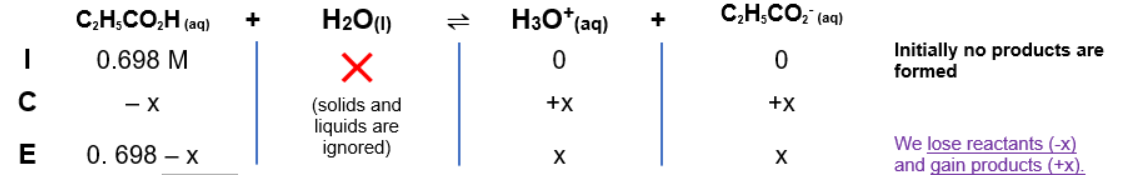# Problem: Propionic acid, C2H5CO2H (Ka = 1.34 × 10−5), is used in the manufacture of calcium propionate, a food preservative. What is the hydronium ion concentration in a 0.698-M solution of C2H5CO2H?

###### FREE Expert Solution

Dissociation: C2H5CO2H (aq) + H2O(l)  H3O+(aq) + C2H5CO2- (aq)

ICE CHART:Ka expression:

$\overline{){{\mathbf{K}}}_{{\mathbf{a}}}{\mathbf{=}}\frac{\mathbf{products}}{\mathbf{reactants}}{\mathbf{=}}\frac{\mathbf{\left[}{\mathbf{H}}_{\mathbf{3}}{\mathbf{O}}^{\mathbf{+}}\mathbf{\right]}\mathbf{\left[}{\mathbf{C}}_{\mathbf{2}}{\mathbf{H}}_{\mathbf{5}}{{\mathbf{CO}}_{\mathbf{2}}}^{\mathbf{-}}\mathbf{\right]}}{\mathbf{\left[}{\mathbf{C}}_{\mathbf{2}}{\mathbf{H}}_{\mathbf{5}}{\mathbf{CO}}_{\mathbf{2}}\mathbf{H}\mathbf{\right]}}}$

91% (227 ratings)###### Problem Details

Propionic acid, C2H5CO2H (Ka = 1.34 × 10−5), is used in the manufacture of calcium propionate, a food preservative. What is the hydronium ion concentration in a 0.698-M solution of C2H5CO2H?

Frequently Asked Questions

What scientific concept do you need to know in order to solve this problem?

Our tutors have indicated that to solve this problem you will need to apply the Weak Acids concept. If you need more Weak Acids practice, you can also practice Weak Acids practice problems.

What professor is this problem relevant for?

Based on our data, we think this problem is relevant for Professor Jones' class at Saint Louis Community College.

What textbook is this problem found in?

Our data indicates that this problem or a close variation was asked in Chemistry - OpenStax 2015th Edition. You can also practice Chemistry - OpenStax 2015th Edition practice problems.